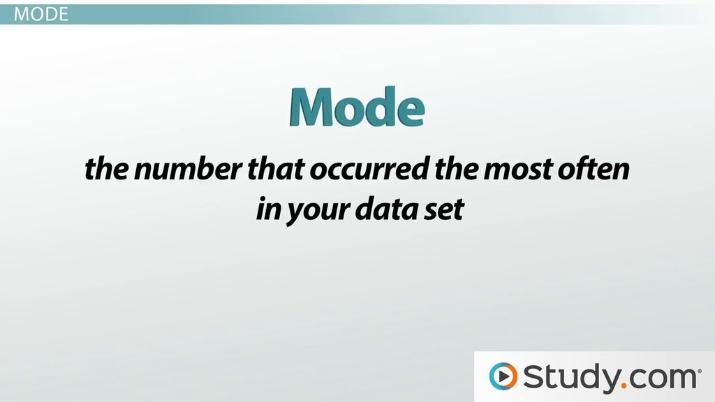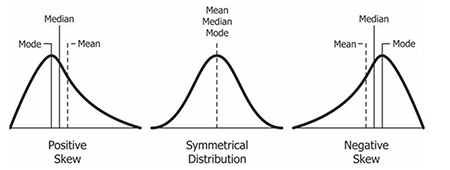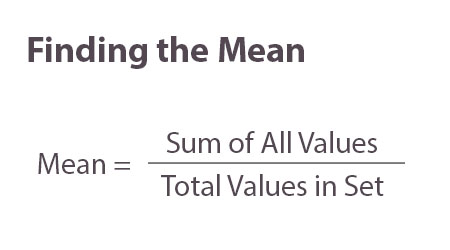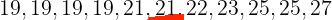# What are the Measures of Central Tendancy?

• Author
Danielle Wilson

Danielle is a certified secondary mathematics, technology, and special education teacher. She holds a BFA in graphic design and Masters degrees in Education, Special Education, and Administration.

• Instructor

Chad has taught Math for the last 9 years in Middle School. He has an M.S. in Instructional Technology and Elementary Education.

What are the measures of central tendency? Learn how to find the mean, median, mode and range in a data set, how each is used in math and view examples. Updated: 06/21/2021

Show

## What are the Measures of Central Tendency?

"Measures of Central Tendency" is one of the most important concepts in the study of descriptive statistics, a type of statistics used to describe and summarize values in a data set. Measures of central tendency do not provide information about the individual values of a data set. Rather, it is a set of numerical values that best represent or summarize the middle of the data set. The following measures can be used to determine the central tendency:

• Mean: the average of the individual values of a data set.
• Median: the number in the middle of a data set with an equal number of values higher and lower than it.
• Mode: the number or value that occurs the most in a data set.

Range is often included when finding the measures of central tendency. However, the range is actually a measure of variation. A measure of variation describes the variability of the data-- or how spread out the data is in a data set.An error occurred trying to load this video.

Try refreshing the page, or contact customer support.

Coming up next: Calculating the Standard Deviation

### You're on a roll. Keep up the good work!

Replay
Your next lesson will play in 10 seconds
• 0:37 Mean
• 1:25 Median
• 4:09 Mode
• 5:27 Range
• 6:05 Example
• 7:51 Lesson Summary
Save Save

Want to watch this again later?

Timeline
Autoplay
Autoplay
Speed Speed

## What are Mean, Median, Mode, and Range Used for?

The mean does not always provide the best measure of central tendency for a data set. When the data is in a symmetrical distribution, or bell-curve, the mean, median, and mode are all at the center of the distribution. If a data set contains outliers, or values that are significantly higher or lower than the rest of the values in the data set, it can look skewed when represented on a graph. A skewed distribution is when the tail of a distribution on a graph is longer than the other. If the tail is longer towards the negative side of a number line, it is left-skewed. If the tail is longer on the positive side of a number line, it is right-skewed. The more a distribution is skewed, either right or left, the less accurate the mean.Unlike the mean, the median is not calculated using all of the numbers in the data set, so the median is not impacted by extreme outliers or heavily skewed distributions.

The mode provides the value that occurs most frequently within a data set. The mode is frequently used with data sets that include categorical data, or data that can be organized into groups, but does not have mathematical meaning. Zip codes and phone numbers are types of numerical data that do not have a mathematical meaning because they do not indicate trends in a data set. Mode is useful for finding the most popular option in a categorical data set like the most favorite color.

Range is a numerical value that represents the spread of a data set. The range can be misleading if there are extreme outliers in the data set.

### How to Find the Mean

In mathematics, finding the mean is synonymous with finding the average of a set of numbers.

To calculate the mean:

1. Determine the total number of values within the dataset.
2. Determine the sum of the values in the data set.
3. Divide the sum of all the values by the total number of values in the dataset.### How to Find the Median

There are two ways to find the median of a data set. The correct method depends on the number of values within the set.

If the data set has an odd quantity of numbers in the set:

1. Organize the values in the set "by magnitude" (from least to greatest)
2. Find the value that is located directly in the middle of the set.

If the data set has an even quantity of numbers in the set:

1. Organize the values in the set by magnitude.
2. Find the two values that are located directly in the middle of the set.
3. Add the the two values together then divide the sum by two.

### How to Find the Mode

The mode is the number that occurs the most in the data set. The mode may not be a unique value if no single number was repeated more than others.

1. Organize the data by groups or magnitude.
2. Identify the value that occurs most frequently within the set.

### How to Find the Range

The range is the numerical difference between the maximum and minimum.

1. Identify the maximum (largest) and minimum (smallest) values of a data set.
2. Subtract the minimum from the maximum.

## Example Using Mean, Median, Mode, and Range

The following is an example of how the mean, median, mode, and range can all be drawn from the same data set.

American Tiger University recorded the following ages for their 2021-2022 academic year roster:

 Student's Name Age Mindy 19 Cameron 22 Tony 19 Keith 23 Lauren 19 Tommy 21 Pilar 25 Mike 19 Carla 27 Kira 25 Marcus 21

### Finding the Mean

To find the mean of the American Tiger University roster, or "data set,":

1. Find the sum of the values of the set.
2. Divide the sum by number of values in the set.

$$\large \frac{19+22+19+23+19+21+25+19+27+25+21}{11} = \frac{240}{11}=21.\bar{81}$$

The average age of a member on the swim team is approximately 21.81.

### Finding the Median

To find the median of the data set:

1. Organize the ages from least to greatest.
2. This set currently has an odd set of values (ages), there will be one median.21 is the single number directly in the center, and the median of the swim team is therefore 21.

To unlock this lesson you must be a Study.com Member.

#### Is mean the same as average?

In mathematics the mean is considered to be the same thing as the average. Both are calculated by finding the sum of the total values in the set, then dividing that number by the number of values within the set.

#### What is range mode and mean?

Range is a measure of variability and represents the spread of the values in a data set. Mode is the number or value that occurs most often in the data set, or the most popular value. Mean is the average, or center of a normal distribution. The mean may not be as accurate in data sets with outliers or skewed distributions.

#### How do you find the mean median and mode?

The mean of a data set can be found by adding the values together and dividing that sum by the total number of values in the set. The median can be found by organizing the values from least to greatest and locating the value that is directly in the center of the set. If there are two values, the mean can be found by adding those two values and dividing that sum by two. The mode is the value that occurs most frequently within a set of data.

### Register to view this lesson

Are you a student or a teacher?